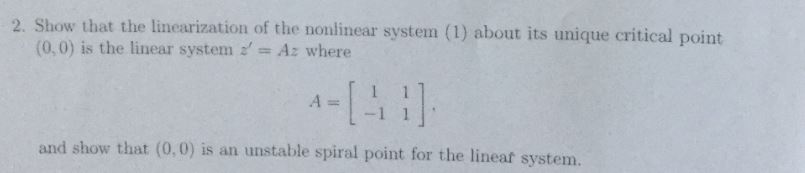# 2. Showthat the linearization of the(0,0) is the linear system Az wherenonlinear system (1) about its unique critical pointand show that (0,0) is an unstable spiral point for the lineaf systenm.

Question
30 views

Pls explain to me step by step. Pls dont skip ay steps. thankshelp_outlineImage Transcriptionclose2. Show that the linearization of the (0,0) is the linear system Az where nonlinear system (1) about its unique critical point and show that (0,0) is an unstable spiral point for the lineaf systenm. fullscreen
check_circle

Step 1

Suppose P=(x0,y0) is an critical point of the system x\'=f(x,y) , y\'=g(x,y),

and that f and g are differentiable at P.

The linearization of the system at P is the linear system.

Step 2

we have given point P=(0,0).

Step 3

Hence, the linear system...

### Want to see the full answer?

See Solution

#### Want to see this answer and more?

Solutions are written by subject experts who are available 24/7. Questions are typically answered within 1 hour.*

See Solution
*Response times may vary by subject and question.
Tagged in

### Math